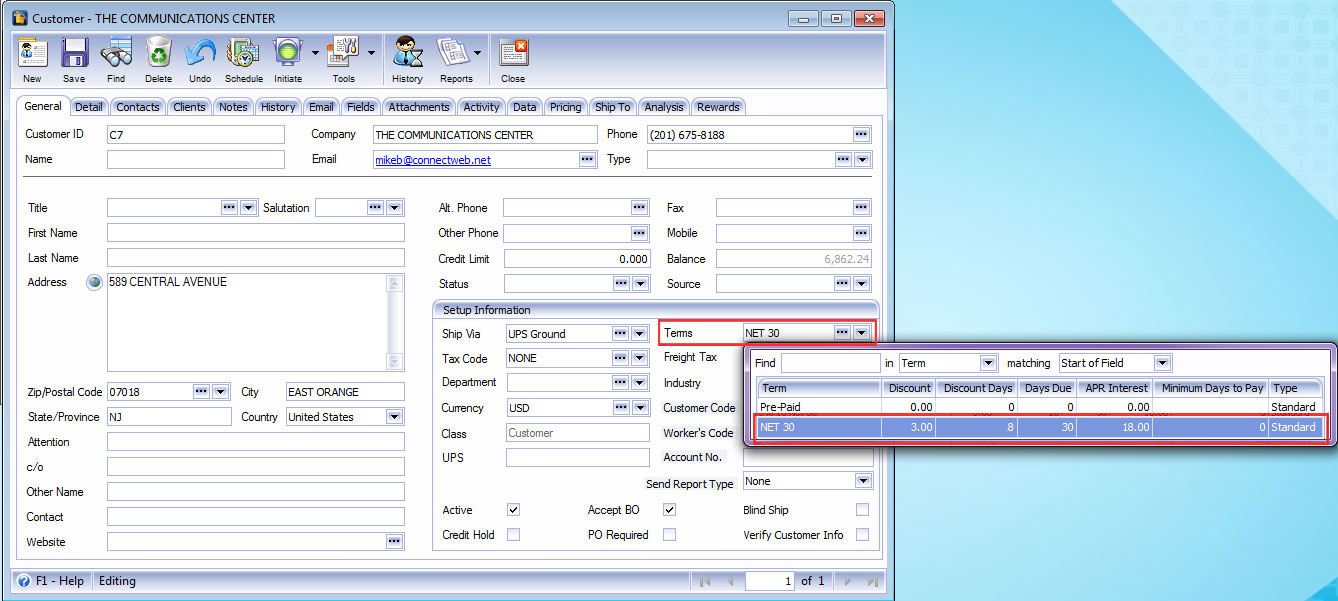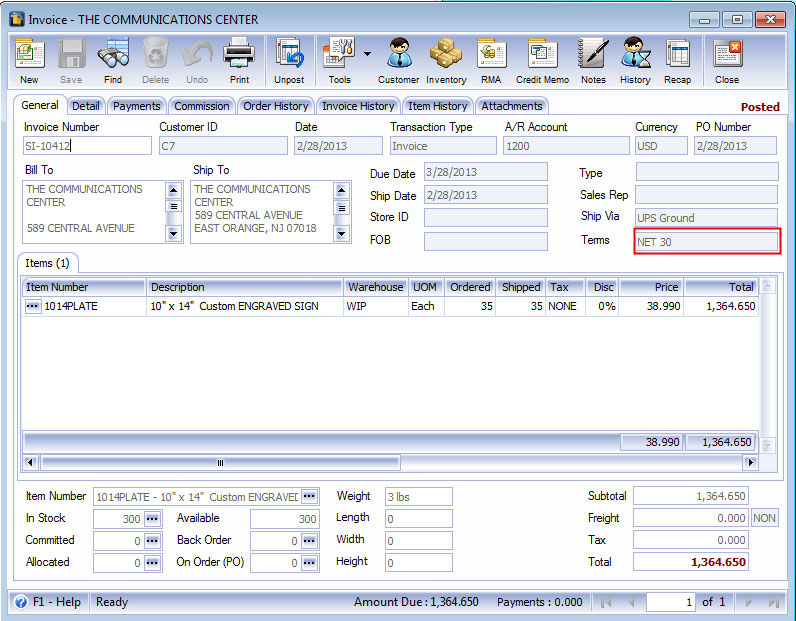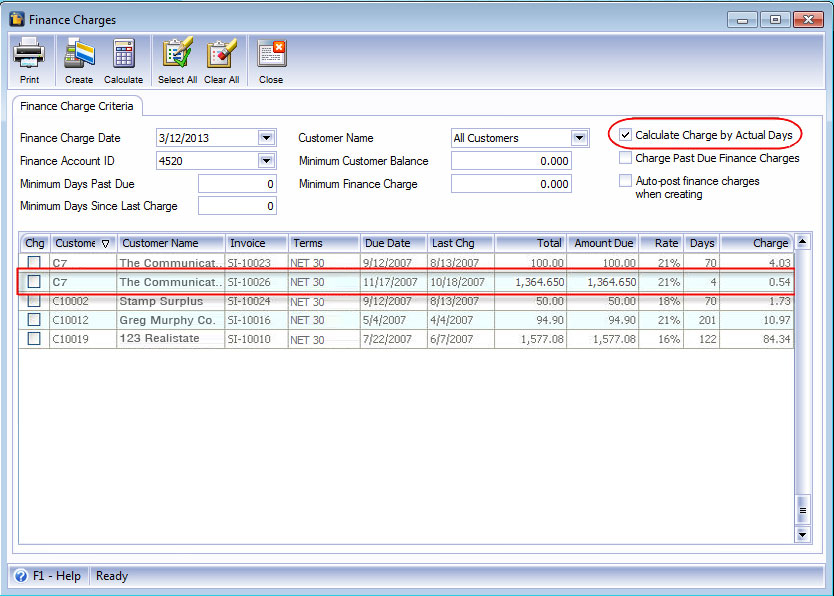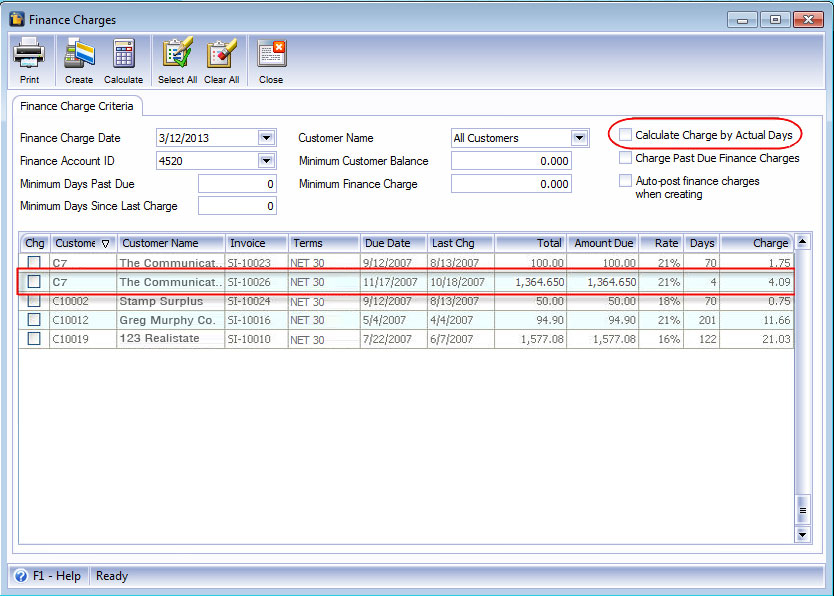﻿ Finance Charge

Finance Charge

Setup a term for a Customer. Terms here is 3% 8 Net 30 which means that Customer C7 (Communication Center) will get 3% discount if she pays it within 8 days. If she pays it beyond 8 days but within 30 days, she gets the regular price. After 30 days, she will incur interest of 18%.Take note of the date of this invoice. This will be your basis of computing Finance Charge. In this example, the invoice date is 2/28/2013. The invoice due date is 3/28/2013 and this is 30 days after your invoice date. After this date, C7 will start incurring finance charges.

Assume that it is 3/21/2013. When you open the Finance Charge form, it will then display this invoice. Here are the examples (see screen shots below) to distinguish the result when using and not using Actual days.

1. If Calculate Charge by Actual Days is checked (Remember that actual days use 365 as denominator)

Formula:

Finance Charge = Invoice Total x [(No. of Days/Actual Days) x Rate]Below is the computation for this example

Finance Charge = Invoice Total x [(4/365) x 21%]

= 233.85 x 0.0023

= 0.54

2. If Calculate Charge by Actual Days is not checked:

Formula:

Finance Charge = Invoice Total x [(No. of Months/12 Months) x Rate]Finance Charge = Invoice Total x [(1/12) x 21%]

= 233.85 x 0.0175

= 4.09